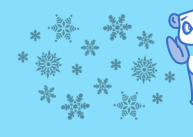Polar calculation ! ... What is the value of each animal ? (7 - 8 years old)
+++= 8
=  ?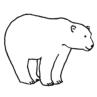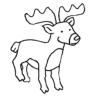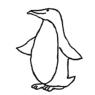54 :
= 3 x=  ?+ (3 x)
=
x=  ?x=+ 22=  ?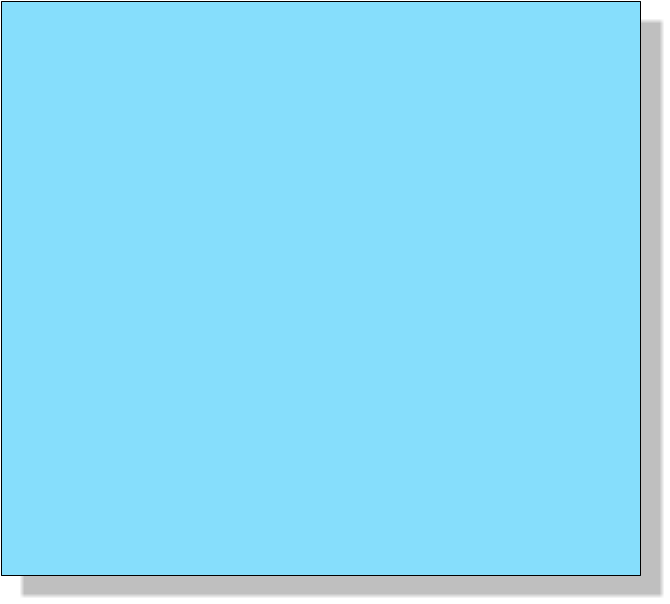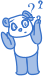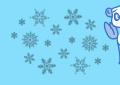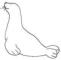Polar calculation ! ... What is the value of each animal ? (7 - 8 years old)
+++= 8
=  ?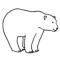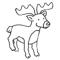54 :
= 3 x=  ?+ (3 x)
=
x=  ?x=+ 22=  ?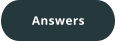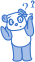Polar calculation ! ... What is the value of each animal ? (7 - 8 years old)
+++= 8
=  ?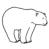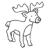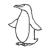54 :
= 3 x=  ?+ (3 x)
=
x=  ?x=+ 22=  ?